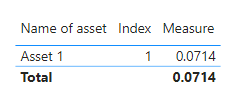cancel
Showing results for
Did you mean:Helper I

## XIIR function in power BI

As we know below is the function for XIRR in power BI:
= XIRR(<tablename>, [Cashflow], [Date])

Consider the below table

 Name of asset Col 1 (Invested amount) Col 2 (Withdrawal amount) Col 3 (Invested date) Col 4 (Withdrawal date) Asset 1 -100000 123000 1 Jan 2020 31 Dec 2022

How do I use the XIRR formula in Power BI for the above table given that we have 2 columns for Cashflow and 2 columns for date in the same row. Please note - I don’t want to unpivot the columns and align the amount and dates columns vertically.
So, the question is - how to use multiple columns for cashflow and multiple columns for dates in the Power BI XIRR formula, something equivalent to what can be easily done in excel e.g. =XIRR (Col1:Col2, Col3:Col4)

1 ACCEPTED SOLUTIONCommunity Support

Hi @ashwinkolte ,

As far as I know, the calculation in Power BI is based on columns. So you need to transform amounts and dates into same columns in Power Query Editor.

``````let
Source = Table.FromRows(Json.Document(Binary.Decompress(Binary.FromText("i45WciwuTi1RMFTSUdI1NAABIMvQyBjKUPBKzFMwMjACcYwNFVxSk0E8I6XYWAA=", BinaryEncoding.Base64), Compression.Deflate)), let _t = ((type nullable text) meta [Serialized.Text = true]) in type table [#"Name of asset" = _t, #" Col 1 (Invested amount)" = _t, #"Col 2 (Withdrawal amount)" = _t, #"Col 3 (Invested date)" = _t, #"Col 4 (Withdrawal date)" = _t]),
#"Changed Type" = Table.TransformColumnTypes(Source,{{"Name of asset", type text}, {" Col 1 (Invested amount)", Int64.Type}, {"Col 2 (Withdrawal amount)", Int64.Type}, {"Col 3 (Invested date)", type date}, {"Col 4 (Withdrawal date)", type date}}),
#"Renamed Columns" = Table.RenameColumns(#"Added Index",{{" Col 1 (Invested amount)", "Invested amount"}, {"Col 2 (Withdrawal amount)", "Withdrawal amount"}, {"Col 3 (Invested date)", "Invested date"}, {"Col 4 (Withdrawal date)", "Withdrawal date"}}),
#"Unpivoted Columns" = Table.UnpivotOtherColumns(#"Renamed Columns", {"Name of asset", "Invested date", "Withdrawal date", "Index"}, "Attribute", "Value"),
#"Unpivoted Columns1" = Table.UnpivotOtherColumns(#"Unpivoted Columns", {"Name of asset", "Index", "Attribute", "Value"}, "Attribute.1", "Value.1"),
#"Replaced Value" = Table.ReplaceValue(#"Unpivoted Columns1"," amount","",Replacer.ReplaceText,{"Attribute"}),
#"Replaced Value1" = Table.ReplaceValue(#"Replaced Value"," date","",Replacer.ReplaceText,{"Attribute.1"}),
#"Filtered Rows" = Table.SelectRows(#"Replaced Value1", each ([Attribute] = [Attribute.1])),
#"Renamed Columns1" = Table.RenameColumns(#"Filtered Rows",{{"Value", "Amount"}, {"Value.1", "Date"}}),
#"Removed Columns" = Table.RemoveColumns(#"Renamed Columns1",{"Attribute.1"})
in
#"Removed Columns"``````

New Table:Measure:

``Measure = XIRR('Table','Table'[Amount],'Table'[Date])``

Result is as below.Best Regards,
Rico Zhou

If this post helps, then please consider Accept it as the solution to help the other members find it more quickly.

3 REPLIES 3Helper I

Can we do this in DAX ?Super User

Hi,

Yes, we can but we will have to unpivot first.

Regards,
Ashish Mathur
http://www.ashishmathur.comCommunity Support

Hi @ashwinkolte ,

As far as I know, the calculation in Power BI is based on columns. So you need to transform amounts and dates into same columns in Power Query Editor.

``````let
Source = Table.FromRows(Json.Document(Binary.Decompress(Binary.FromText("i45WciwuTi1RMFTSUdI1NAABIMvQyBjKUPBKzFMwMjACcYwNFVxSk0E8I6XYWAA=", BinaryEncoding.Base64), Compression.Deflate)), let _t = ((type nullable text) meta [Serialized.Text = true]) in type table [#"Name of asset" = _t, #" Col 1 (Invested amount)" = _t, #"Col 2 (Withdrawal amount)" = _t, #"Col 3 (Invested date)" = _t, #"Col 4 (Withdrawal date)" = _t]),
#"Changed Type" = Table.TransformColumnTypes(Source,{{"Name of asset", type text}, {" Col 1 (Invested amount)", Int64.Type}, {"Col 2 (Withdrawal amount)", Int64.Type}, {"Col 3 (Invested date)", type date}, {"Col 4 (Withdrawal date)", type date}}),
#"Renamed Columns" = Table.RenameColumns(#"Added Index",{{" Col 1 (Invested amount)", "Invested amount"}, {"Col 2 (Withdrawal amount)", "Withdrawal amount"}, {"Col 3 (Invested date)", "Invested date"}, {"Col 4 (Withdrawal date)", "Withdrawal date"}}),
#"Unpivoted Columns" = Table.UnpivotOtherColumns(#"Renamed Columns", {"Name of asset", "Invested date", "Withdrawal date", "Index"}, "Attribute", "Value"),
#"Unpivoted Columns1" = Table.UnpivotOtherColumns(#"Unpivoted Columns", {"Name of asset", "Index", "Attribute", "Value"}, "Attribute.1", "Value.1"),
#"Replaced Value" = Table.ReplaceValue(#"Unpivoted Columns1"," amount","",Replacer.ReplaceText,{"Attribute"}),
#"Replaced Value1" = Table.ReplaceValue(#"Replaced Value"," date","",Replacer.ReplaceText,{"Attribute.1"}),
#"Filtered Rows" = Table.SelectRows(#"Replaced Value1", each ([Attribute] = [Attribute.1])),
#"Renamed Columns1" = Table.RenameColumns(#"Filtered Rows",{{"Value", "Amount"}, {"Value.1", "Date"}}),
#"Removed Columns" = Table.RemoveColumns(#"Renamed Columns1",{"Attribute.1"})
in
#"Removed Columns"``````

New Table:Measure:

``Measure = XIRR('Table','Table'[Amount],'Table'[Date])``

Result is as below.Best Regards,
Rico Zhou

If this post helps, then please consider Accept it as the solution to help the other members find it more quickly.